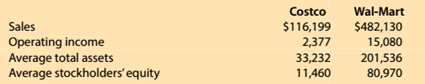Chapter 15, Problem 15.5.1MBA

Chapter
Section
Textbook Problem

Financial leverageCostco Wholesale Corporation (COST) and Wel-Mart Stroes Inc. (WMT)reported the following data (in milllions) for a recent year:1. Compute the return on total assets. Round to one decimal place.

To determine

Concept Introduction:

Return on total Assets:

The Return on total assets is profitability ratio that measures the percentage of profit earned on average assets invested in the business. Return on asset is calculated by dividing the net income by average total assets. The formula to calculate Return on assets is as follows:

Return on assets = Net incomeAverage Total Assets

Note: Average total assets are calculated as an average of beginning and ending total assets. The formula to calculate the average total assets is as follows:

Average total Assets = (Beginning total assets + Ending total assets)2

To Calculate:

The return on total assets

Explanation

The return on total assets is calculated as follows:

 $in Millions Costco Wal-Mart Operating Income (A)$ 2,377

Still sussing out bartleby?

Check out a sample textbook solution.

See a sample solution

The Solution to Your Study Problems

Bartleby provides explanations to thousands of textbook problems written by our experts, many with advanced degrees!

Get Started

PREPARE CHECK AND STUB Based on the following information, prepare a check and stub:

College Accounting, Chapters 1-27 (New in Accounting from Heintz and Parry)

What is managerial accounting

Managerial Accounting: The Cornerstone of Business Decision-Making

Explain why an economys income must equal its expenditure.

Brief Principles of Macroeconomics (MindTap Course List)

CAPITAL BUDGETING CRITERIA Your division is considering two projects. Its WACC is 10%, and the projects after-t...

Fundamentals of Financial Management, Concise Edition (with Thomson ONE - Business School Edition, 1 term (6 months) Printed Access Card) (MindTap Course List)